# Class 11 Maths NCERT Solutions for Chapter 9 Sequences and Series Exercise 9.2### Sequences and Series Exercise 9.2 Solutions

1. Find the sum of odd integers from 1 to 2001.

Solution

The odd integers from 1 to 2001 are 1, 3, 5, …1999, 2001.
This sequence forms an A.P.
Here, first term, a = 1
Common difference, d = 2
Here, a + (n-1)d = 2001
⇒ 1 + (n - 1)(2) = 2001
⇒ 2n - 2 = 2000
⇒ n = 1001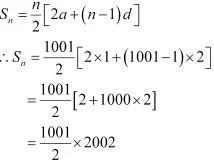= 1001 × 1001
= 1002001
Thus, the sum of odd numbers from 1 to 2001 is 1002001.

2. Find the sum of all natural numbers lying between 100 and 1000, which are multiples of 5.

Solution

The natural numbers lying between 100 and 1000, which are multiples of  5, are 105, 110, .... 995.
Here, a = 105 and d = 5
a + (n - 1)d = 995
⇒ 105 + (n - 1)5  = 995
⇒ (n- 1)5 = 995 - 105 = 890
⇒ n - 1 = 178
⇒ n = 179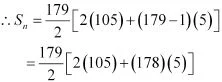= 179[2(105) + (178)(5)]
= (179)(105 + 445)
= (179 × 550)
= 98450
Thus, the sum of all natural numbers lying between 100 and 1000, which are multiples of 5, is 98450.

3. In an A.P, the first term is 2 and the sum of the first five terms is one-fourth of the next five terms. Show that 20th term is –112.

Solution

First term = 2
Let d be the common difference of the A.P.
Therefore, the A.P. is 2, 2 + d, 2 + 2d, 2 + 3d, …
Sum of first five terms = 10 + 10d
Sum of next five terms = 10 + 35d
According to the given condition,
10 + 10d = (1/4)(10 + 35d)
⇒ 40 + 40d = 10 + 35d
⇒ 30 = -5d
⇒ d = -6
∴ a20 = a + (20 - 1)d = 2 + (19)(-6) = 2 - 114 = -112
Thus, the 20th term of the A.P. is -112.

4. How many terms of the A.P. -6, -11/2, -5... are needed to give the sum –25?

Solution

Let the sum of n terms of the given A.P. be  -25.
It is known that, Sn = (n/2) [2a + (n - 1)d], where n = number of terms, a = first term, and d = common difference
Here, a = -6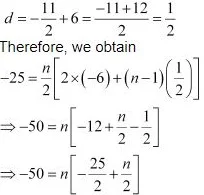⇒-100 = n(-25 + n)
⇒ n2 - 25n + 100 = 0
⇒ n2 - 5n - 20n + 100 = 0
⇒ n(n -5) - 20(n - 5) = 0
⇒ n = 20 or 5

5. In an A.P., if pth term is 1/q and qth  term is 1/p, prove that the sum of first pq terms is 1/2 (pq + 1) where p ≠ q.

Solution

It is known that the general term of an A.P. is an = a + (n – 1)d
∴ According to the given information,
pth  term = ap = a + (p - 1)d = 1/q  ...(1)
qth  term = aq = a + (q - 1)d = 1/p  ...(2)
Subtracting (2) from (1), we obtain
(p - 1)d - (q - 1)d = 1/q - 1/p
⇒ (p - 1 - q + 1)d = (p -q)/pq
⇒ (p -q )d = (p - q)/pq
⇒ d = 1/pq
Putting the value of d in (1), we obtain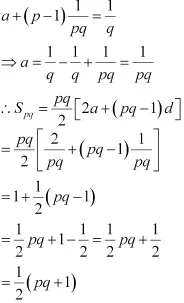Thus, the sum of first pq terms of the A.P. is 1/2(pq + 1).

6. If the sum of a certain number of terms of the A.P. 25, 22, 19, … is 116. Find the last term

Solution

Let the sum of n terms of the given A.P. be 116.
Sn = (n/2) [2a + (n - 1)d]
Here, a = 25 and d = 22 - 25 = -3
∴ Sn = (n/2) [2× 25 + (n - 1)(-3)]
⇒  116 = n/2[50 - 3n + 3]
⇒  232 = n (53 -3n) = 53n - 3n2
⇒ 3n2 - 53n + 232 = 0
⇒ 3n2 - 24n - 29n + 232 = 0
⇒ 3n(n - 8) - 29(n - 8) = 0
⇒ (n -8)(3n - 29) = 0
⇒ n = 8 or n = 29/3
However, n cannot be equal to 29/3 . Therefore, n = 8
∴ a8 = Last term = a + (n - 1)d = 25 + (8 -1)(-3)
= 25 + (7)(-3) = 25 - 21
= 4
Thus, the last term of the A.P. is 4.

7. Find the sum to n terms of the A.P., whose kth term is 5k + 1.

Solution

It is given that the kth term of the A.P. is 5k + 1.
kth term = ak = + (k – 1)d
∴ + (k – 1)d = 5k + 1
a + kd – d = 5k + 1
Comparing the coefficient of k, we obtain d = 5
– = 1
⇒ a – 5 = 1
⇒ a = 6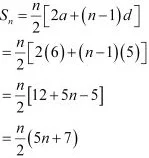8. If the sum of n terms of an A.P. is (pn qn2), where p and q are constants, find the common difference.

Solution

It is known that, Sn = (n/2) [2a + (n - 1)d]
According to the given condition,
n/2 [2a + (n - 1)d] = pn + qn2
⇒ n/2 [2a + nd - d] = pn + qn2
⇒ na + n2 (d/2) - n .(d/2) = pn + qn2
Comparing the coefficients of n2 on both sides, we obtain
d/2 = q
∴ d = 2q
Thus, the common difference of the A.P. is 2q.

9. The sums of n terms of two arithmetic progressions are in the ratio 5n + 4: 9n + 6. Find the ratio of their 18th terms

Solution

Let a1a2, and d1dbe the first terms and the common difference of the first and second arithmetic progression respectively.
According to the given condition,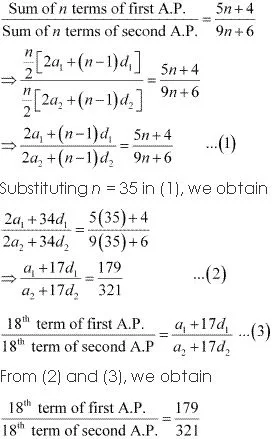Thus, the ratio of 18th term of both the A.P. is 179 : 321.

10. If the sum of first p terms of an A.P. is equal to the sum of the first q terms, then find the sum of the first (p + q) terms.

Solution

Let a and d be the first term and the common difference of the A.P. respectively.
Here,
Sp = p/2 [2a + (p -1)d]
Sq = q/2 [2a + (q -1)d]
According to the given condition,
p/2 [2a + (p - 1)d] = q/2 [2a + (q - 1)d]
⇒ p[2a + (p - 1)q] = q[2a + (q - 1)d]
⇒ 2ap + pd(p -1) = 2aq + qd(q - 1)
⇒ 2a(p -q) + d[p(p -1) - q(q - 1)] = 0
⇒ 2a(p - q) +d [p2 - p - q2 + q] = 0
⇒ 2a(p - q) +d [(p -q)(p +q) - (p -q)] = 0
⇒ 2a(p - q) +d [(p -q)(p + q - 1)] = 0
⇒ 2a + d(p + q - 1) = 0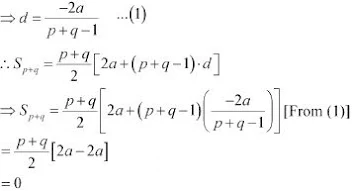Thus, the sum of the first (p + q) terms of the A.P. is 0.

11. Sum of the first p, q and r terms of an A.P. are a, b and c, respectively.
Prove that a/q (q - r) + b/q (r - p) + c/r (p - q) = 0

Solution

Let a1 and d be the first term and the common difference of the A.P. respectively.
According to the given information,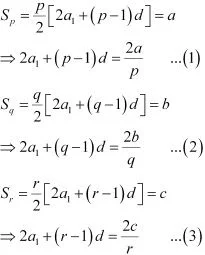Subtracting (2) from (1), we obtain
(p - 1)d - (q - 1)d = 2a/p - 2b/q
⇒ d(p - 1 - q + 1) = (2aq - 2bp)/pq
⇒ d(p -q) = (2aq - 2bp)/pq
⇒ d = 2(aq - bp)/pq(p -q)  ...(4)
Subtracting (3) from (2), we obtain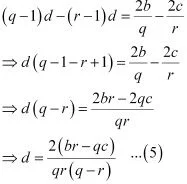Equating both the values of d obtained in (4) and (5), we obtain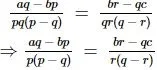⇒ r(q - r)(aq - bp) = p(p - q)(br - qc)
⇒ r(aq - bp)(q - r) = p(br - qc)(p - q)
⇒ (aqr - bpr)(q - r) = (bpr - cpq)(p - q)
Dividing both sides by pqr, we obtain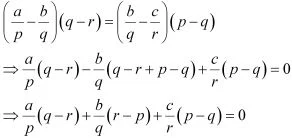Thus, the given result is proved.

12. The ratio of the sums of m and n terms of an A.P. is m2: n2. Show that the ratio of mth and nth term is (2m – 1): (2n – 1).

Solution

Let a and d be the first term and the common difference of the A.P. respectively.
According to the given condition,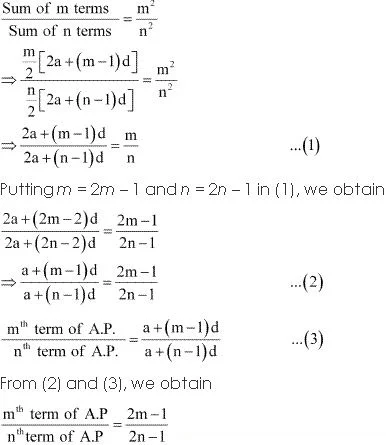Thus, the given result is proved.

13. If the sum of n terms of an A.P. is 3n2 + 5n and its mth term is 164, find the value of m.

Solution

Let a and b be the first term and the common difference of the A.P. respectively.
am = a + (m – 1)d = 164 …(1)
Sum of n terms,
Sn = (n/2) [2a + (n - 1)d]
Here,
n/2 [2a + nd - d] = 3n2 + 5n
⇒ na + (d/2)n2  - (d/2)n = 3n2 + 5n
⇒ (d/2)n2 + (a - d/2)n = 3n2 + 5n
Comparing the coefficient of n2 on both sides, we obtain
d/2 = 3
⇒ d = 6
Comparing the coefficient of n on both sides, we obtain
a - d/2 = 5
⇒ a - 3 = 5
⇒ a = 8
Therefore, from (1), we obtain
8 + (m - 1)6 = 164
⇒ (m - 1)6 = 164 - 8 = 156
⇒ m - 1 =26
⇒ m = 27
Thus, the value of m is  27.

14. Insert five numbers between 8 and 26 such that the resulting sequence is an A.P.

Solution

Let A1, A2, A3, A4, and A5 be five numbers between 8 and 26 such that
8, A1, A2, A3, A4, A5, 26 is an A.P.
Here, = 8, = 26, n = 7
Therefore, 26 = 8 + (7 – 1) d
⇒ 6d = 26 – 8 = 18
⇒ = 3
A1 = a + d = 8 + 3 = 11
A2 = a + 2d = 8 + 2 × 3 = 8 + 6 = 14
A3 = a + 3d = 8 + 3 × 3 = 8 + 9 = 17
A4 = a + 4= 8 + 4 × 3 = 8 + 12 = 20
A5 = a + 5d = 8 + 5 × 3 = 8 + 15 = 23
Thus, the required five numbers between 8 and 26 are 11, 14, 17, 20, and 23.

15. If  (an + bn )/(an-1 + bn-1 ) is the A.M. between a and b, then find the value of n.

Solution

A.M. of a and b = (a + b)/2
According to the given condition,
(a + b)/2 = (an + bn)/(an-1 + bn-1
⇒ (a + b)(an-1 + bn-1) = 2(an + bn
⇒ an + abn-1 + ban-1 + bn = 2an + 2bn
⇒ abn-1 + an-1 b = an + bn
⇒ abn-1 - bn = an - an-1 b
⇒ bn-1 (a - b) = an-1 (a - b)
⇒ bn-1 = an-1
⇒ (a/b)n-1 = 1 = (a/b)n
⇒ n - 1 = 0
⇒ n = 1

16. Between 1 and 31, m numbers have been inserted in such a way that the resulting sequence is an A.P. and the ratio of 7th and (m – 1)th numbers is 5:9. Find the value of m.

Solution

Let A1, A2, … Am be m numbers such that 1, A1, A2, … Am, 31 is an A.P.
Here, a = 1, b = 31, n = m + 2
∴ 31 = 1 + (m + 2 – 1) (d)
⇒ 30 = (m + 1) d
⇒ d = 30/(m + 1)  ...(1)
A1 = a + d
A2 = a + 2d
A3 = a + 3d
∴ A7 = a + 7d
Am–1 = a + (m – 1) d
According to the given condition,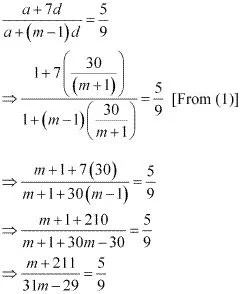⇒ 9m + 1899 = 155m - 145
⇒ 155m - 9m = 1899 + 145
⇒ 146m = 2044
⇒ m = 14
Thus, the value of m is  14.

17. A man starts repaying a loan as first installment of Rs. 100. If he increases the installment by Rs 5 every month, what amount he will pay in the 30th installment?

Solution

The first installment of the loan is Rs 100.
The second installment of the loan is Rs 105 and so on.
The amount that the man repays every month forms an A.P.
The A.P. is 100, 105, 110, …
First term, a = 100
Common difference, d = 5
A30 = a + (30 – 1)d
= 100 + (29) (5)
= 100 + 145
= 245
Thus, the amount to be paid in the 30th installment is Rs 245.

18. The difference between any two consecutive interior angles of a polygon is 5°. If the smallest angle is 120°, find the number of the sides of the polygon.

Solution

The angles of the polygon will form an A.P. with common difference d as 5° and first term a as 120°.
It is known that the sum of all angles of a polygon with n sides is 180° (n – 2).
∴ Sn = 180° (n - 2)
⇒  n/2 [2a + (n - 1)d]  = 180° (n - 2)
⇒  n/2 [2a + (n - 1)d]  = 180° (n - 2)
⇒  n [240 + (n - 1)5]  = 360(n - 2)
⇒ 240 n + 5n2 - 5n = 360n - 720
⇒ 5n2 + 235n - 360n + 720 = 0
⇒ 5n2 - 125n + 720 = 0
⇒ n2 - 25n + 144 = 0
⇒ n2 - 16n - 9n + 144 = 0
⇒ n(n - 16) - 9(n - 16) = 0
⇒ (n - 9)(n - 16) = 0
⇒ n = 9 or 16